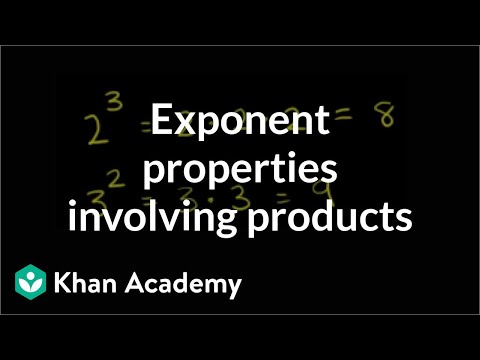Video

# Exponent properties with quotients (Full video)

Khan Academy

## Supporting grades: 8

Description: Learn how to simplify expressions like (5^6)/(5^2). Also learn how 1/(a^b) is the same as a^(-b). Towards the end of the video, we practice simplifying more complex expressions like [25x(y^6)]/[20(y^5)(x^2)]. Created by Sal Khan and CK-12 Foundation. Let's say I were to ask you what 5 to the sixth power divided by 5 to the second power is? Well, we can just go to the basic definition of what an exponent represents and say 5 to the sixth power, that's going to be 5 times 5 times 5 times 5 times 5 times 5. So this really was equal to 5 to the sixth power minus 2.

You must log inorsign upif you want to:*

*Teacher Advisor is 100% free.

### Other videos you might be interested in### Exponent properties with parentheses (Full video)

#### Khan Academy